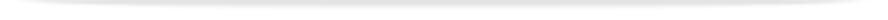units

MTH1030

Faculty of Scienceprint version

This unit entry is for students who completed this unit in 2016 only. For students planning to study the unit, please refer to the unit indexes in the the current edition of the Handbook. If you have any queries contact the managing faculty for your course or area of study.

MTH1030 - Techniques for modelling

6 points, SCA Band 2, 0.125 EFTSL

Refer to the specific census and withdrawal dates for the semester(s) in which this unit is offered.

Faculty

Science

Organisational Unit

School of Mathematical Sciences

Coordinator(s)

Offered

Clayton

• First semester 2016 (Day)
• Second semester 2016 (Day)

Synopsis

Solution of systems of linear equations using Gaussian elimination; matrices, determinants, eigenvalues and eigenvectors; introduction to vectors; methods of integration - substitutions and integration by parts; solution of first-order ordinary differential equations - separable, use of integrating factor; solution of second-order linear ordinary differential equations with constant coefficients and applications; Taylor series and series convergence; the remainder term.

Outcomes

On completion of this unit students will be able to:

1. Understand the basic concepts of linear algebra, recognise and manipulate elements of vector spaces;

1. Formulate and solve equations involving vectors and matrices, including for three-dimensional geometry;

1. Identify and evaluate improper integrals;

1. Solve simple first and second order differential equations, and formulate them for applications to physical systems;

1. Compute Taylor series expansions, with remainder, for functions of one variable;

1. Apply Taylor series and l'Hopital's rule to compute limits;

1. Understand and compute the convergence properties of infinite series;

1. Provide written reports that contain complete mathematical arguments.

Assessment

Final examination (3 hours): 70%
Assignments and tests: 30%

Three 1-hour lectures and one 2-hour computer laboratory per week

Prerequisites

MTH1020 or VCE Specialist Mathematics units 3 and 4 with a study score of at least 30

Prohibitions

ENG1005, ENG1091, MTH1035